# Tangent linear hard sphere chains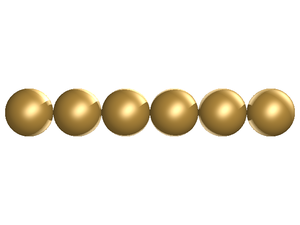Example of the tangent linear hard sphere chain model, with m=6.

The tangent linear hard sphere chain model consists of, as the name suggests, chains of varying monomer length (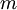$m$), placed tangentially in a linear configuration. Each monomer is a hard sphere model and each molecule is rigid.

## Liquid crystals

For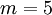$m=5$ smectic-A phase becomes stable. For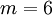$m=6$ the nematic phase becomes stable .

## Excluded volume

The excluded volume for this model is given by ( Eq. 30):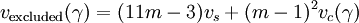$v_{\mathrm {excluded}}(\gamma)= (11m -3)v_s + (m-1)^2 v_c(\gamma)$

where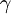$\gamma$ is the relative orientation of the principal axes of the molecules. See Ref. 2 for details of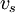$v_s$ and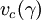$v_c(\gamma)$.